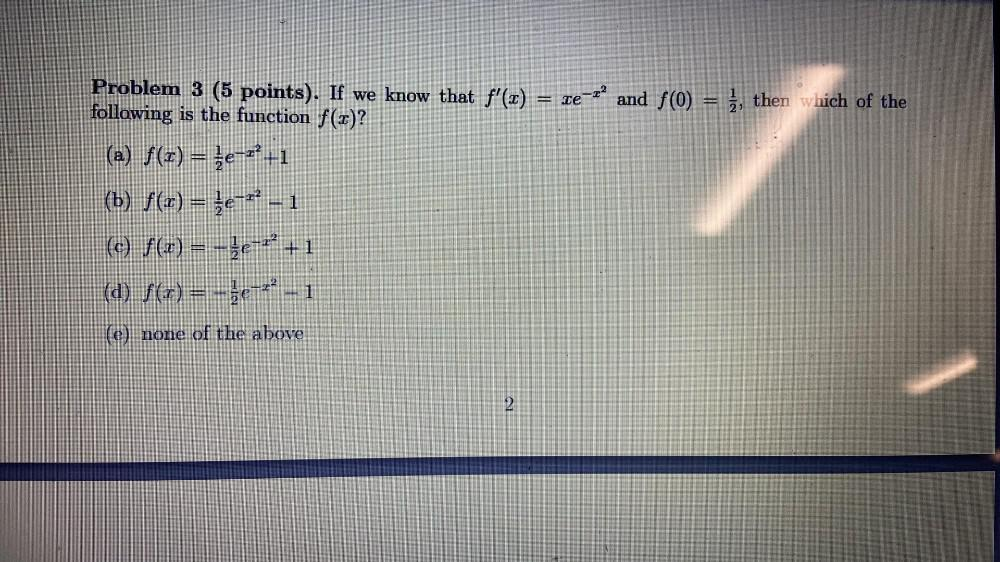Question:

# ET Problem 3 (5 points). If we know that f'(x) = xe-rand f(0) = è, then which of the following is the function f(x)? (a) f(x) =ET Problem 3 (5 points). If we know that f'(x) = xe-rand f(0) = è, then which of the following is the function f(x)? (a) f(x) = {e-r-1 (b) f(1) = {e-r? - 1 (c) f(x) = -je-r' +1 (a) f(x) = -3e-4-1 (e) none of the above 2